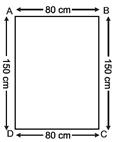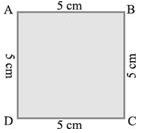Courses

# Test: Mensuration - 2

## 20 Questions MCQ Test Mathematics (Maths) Class 6 | Test: Mensuration - 2

Description
This mock test of Test: Mensuration - 2 for Class 6 helps you for every Class 6 entrance exam. This contains 20 Multiple Choice Questions for Class 6 Test: Mensuration - 2 (mcq) to study with solutions a complete question bank. The solved questions answers in this Test: Mensuration - 2 quiz give you a good mix of easy questions and tough questions. Class 6 students definitely take this Test: Mensuration - 2 exercise for a better result in the exam. You can find other Test: Mensuration - 2 extra questions, long questions & short questions for Class 6 on EduRev as well by searching above.
QUESTION: 1

Solution:
QUESTION: 2

Solution:
QUESTION: 3

### If the perimeter of a square is 16 cm, then its area is

Solution:
QUESTION: 4

The side of a square is 8 cm. If its side is doubled, then its new perimeter is

Solution:
QUESTION: 5

The length and breadth of a rectangle are 10 cm and 8 cm respectively. If its length is doubled, then its new area is

Solution:
QUESTION: 6

Area of a rectangle = ________

Solution:
QUESTION: 7

Find the area of a square plot of side 7 m.

Solution:

Side of Square = a2

(7)2

= 49 m2

QUESTION: 8

__________ is the distance covered along the boundary forming a closed figure when you go round the figure once.

Solution:
QUESTION: 9

Perimeter of a square = ________ × length of a side

Solution:
QUESTION: 10

Meera went to a park 150 m long and 80 m wide. She took one complete round on its boundary. What is the distance covered by her?

Solution:Let ABCD is a park whose lengths are BC, AD and widths are AB, CD, respectively. Hence, AB = CD = 80 m. and BC = DA = 150m Now, the sum of the lengths of four sides

=AB + BC + CD + DA

= 80 m + 150 m + 80 m + 150 m

= (80 + 150 + 80 + 150) m

=460 m

Perimeter of the park = sum of the lengths of four sides of the park = 460 m

Hence, distance covered by Meera is 460 m.

QUESTION: 11

A room is 4 m long and 3 m 50 cm wide. How many square metres of carpet is needed to cover the floor of the room?

Solution:
QUESTION: 12

Find the area of square whose perimeter is 48cm

Solution:
QUESTION: 13

Find the perimeter of a regular pentagon with each side measuring 3 cm.

Solution:
QUESTION: 14

What is the perimeter of the following figure ?Solution:
QUESTION: 15

Find the perimeter of an isosceles triangle with equal sides 8 cm each and third side 6 cm.

Solution:
QUESTION: 16

Perimeter of a rectangle = _________ × (length + breadth)

Solution:
QUESTION: 17

Perimeter of an __________ = 3 × length of a side

Solution:
QUESTION: 18

A farmer has a rectangular field of length and breadth 240 m and 180 m respectively. He wants to fence it with 3 rounds of rope. What is the total length of rope he must use?

Solution:
QUESTION: 19

If the area of rectangle increases from 2 cm2 to 4 cm2 the perimeter will

Solution:
QUESTION: 20

Which figure encloses more area: a square of side 2 cm ; a rectangle of side 3 cm and 2 cm ;An equilateral triangle of side 4 cm?

Solution: